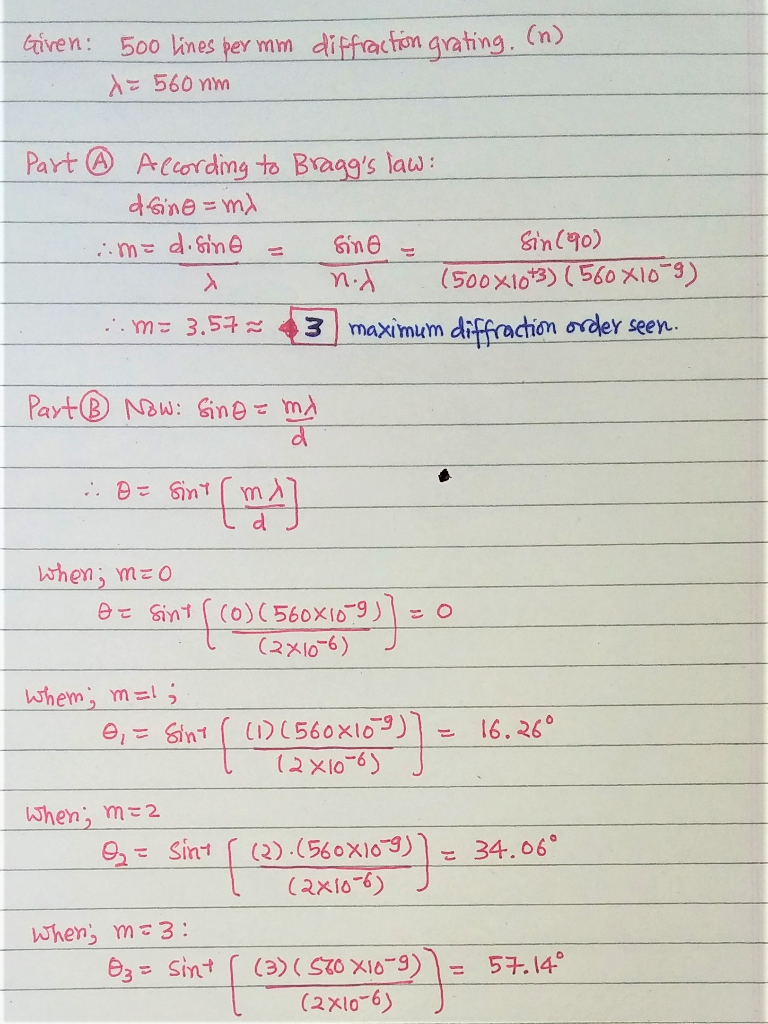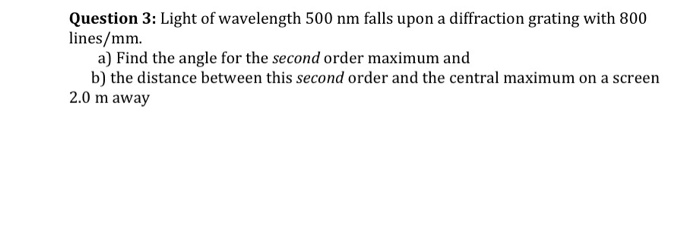Question

# A 500 lines per mm diffraction grating is illuminated by light of wavelength 560 nm ....

 A 500 lines per mm diffraction grating is illuminated by light of wavelength 560 nm .

Part A

What is the maximum diffraction order seen

Part B:

What is the angle of each diffraction order starting from zero diffraction order to the maximum visible diffraction order?Alright Dude, If that worked for you... dont forget to give THUMBS UP.(that will work for me!)
atleast before giving downvote.
and, Thanks for using homeworklib- Smarter way to study.

#### Earn Coins

Coins can be redeemed for fabulous gifts.

Similar Homework Help Questions
• ### A 500 lines per mmmm diffraction grating is illuminated by light of wavelength 630 nm. 1....

A 500 lines per mmmm diffraction grating is illuminated by light of wavelength 630 nm. 1. What is the maximum diffraction order seen? Express your answer as an integer. 2. What is the angle of each diffraction order starting from zero diffraction order to the maximum visible diffraction order? Enter your answers in degrees in ascending order separated by commas.

• ### A 500 lines per mm diffraction grating is illuminated by light of wavelength 640 nm .

A 500 lines per mm diffraction grating is illuminated by light of wavelength 640 nm . For the steps and strategies involved in solving a similar problem, you may view a Video Tutor Solution.Part AWhat is the maximum diffraction order seen? Express your answer as an integer. Part B What is the angle of each diffraction order starting from zero diffraction order to the maximum visible diffraction order?

• ### A 500 lines per mm diffraction grating is illuminated by light of wavelength 510...continues

A 500 lines per mm diffraction grating is illuminated by light of wavelength 510 nm. What is the angle of each diffraction order?

• ### A diffraction grating with an unknown number of lines per mm, is illuminated by light at...

A diffraction grating with an unknown number of lines per mm, is illuminated by light at a wavelength l = 633 mm, and the first order diffraction pattern is seen at an angle β= 45.OO. Light of a second wavelength is shone on to the grating, whose second order diffraction pattern is seen at l = 62.00. What is the wavelength of the second beam?

• ### A diffraction grating with 610 lines per mm is illuminated with light of wavelength 520 nm...

A diffraction grating with 610 lines per mm is illuminated with light of wavelength 520 nm . A very wide viewing screen is 2.0 m behind the grating. Part A What is the distance between the two m=1 fringes? Express your answer in meters. ΔyΔ y = nothing m Request Answer Part B How many bright fringes can be seen on the screen? Express your answer as an integer.

• ### A 480 lines/mm diffraction grating is illuminated by light of wavelength 510 nm . How many...

A 480 lines/mm diffraction grating is illuminated by light of wavelength 510 nm . How many bright fringes are seen on a 4.0-m-wide screen located 2.1 m behind the grating?

• ### A diffraction grating with 600 lines/mm is illuminated with light of wavelength 510 nm. A very...

A diffraction grating with 600 lines/mm is illuminated with light of wavelength 510 nm. A very wide viewing screen is 4.2 m behind the grating. Part A What is the distance between the two m = 1 bright fringes? Express your answer with the appropriate units. Δy = SubmitMy AnswersGive Up Part B How many bright fringes can be seen on the screen? N = SubmitMy AnswersGive Up

• ### A 500 line/mm diffraction grating is illuminated by light of wavelength 510nm. how many diffraction orders...

A 500 line/mm diffraction grating is illuminated by light of wavelength 510nm. how many diffraction orders are seen, and what is the angle of each?

• ### Question 3: Light of wavelength 500 nm falls upon a diffraction grating with 800 lines/mm. a)...Question 3: Light of wavelength 500 nm falls upon a diffraction grating with 800 lines/mm. a) Find the angle for the second order maximum and b) the distance between this second order and the central maximum on a screen 2.0 m away

• ### A 4.4-cm-wide diffraction grating has 2400 slits. It is illuminated by light of wavelength 530 nm...

A 4.4-cm-wide diffraction grating has 2400 slits. It is illuminated by light of wavelength 530 nm . What is the angle (in degrees) of the first diffraction order? What is the angle (in degrees) of the second diffraction order?